# Signals and Systems part 2 solutions

23 de Mar de 2023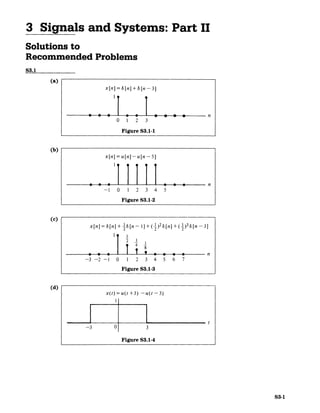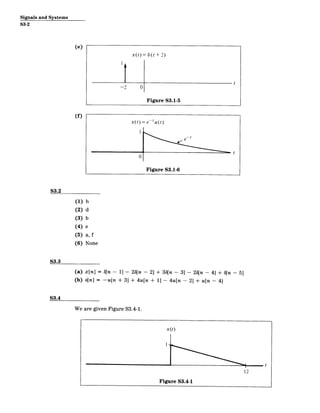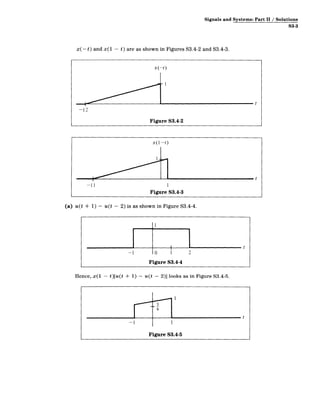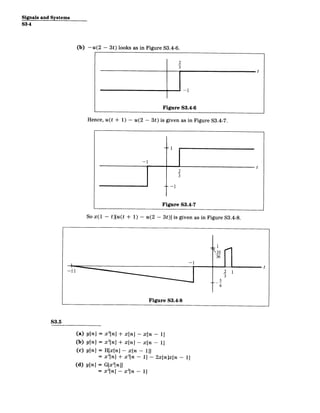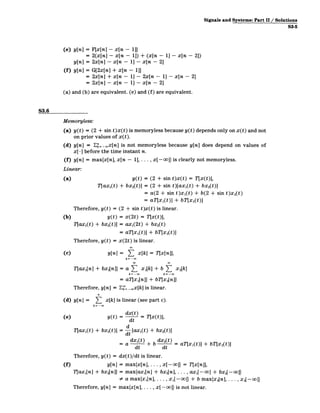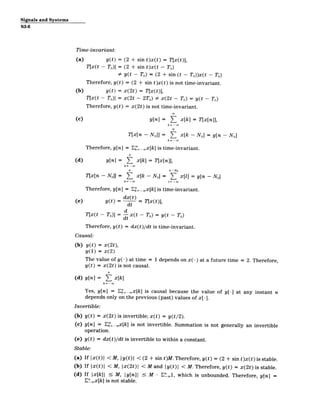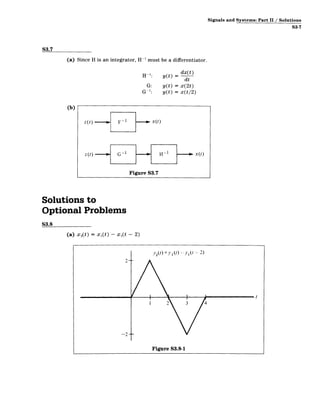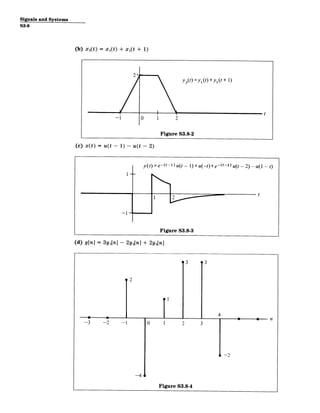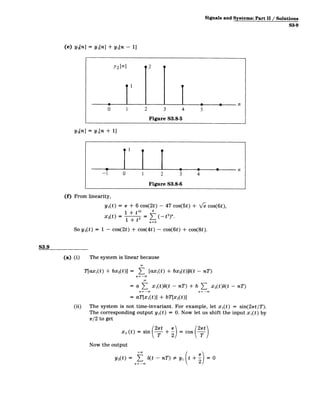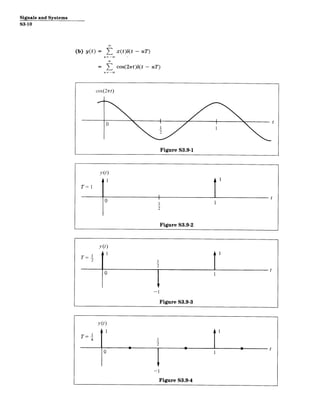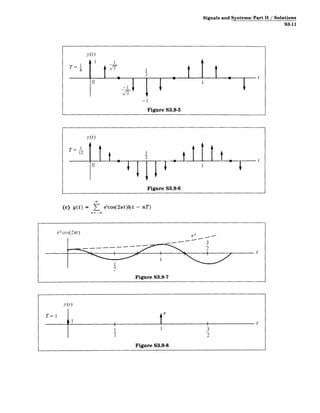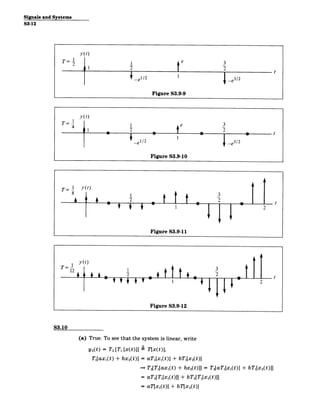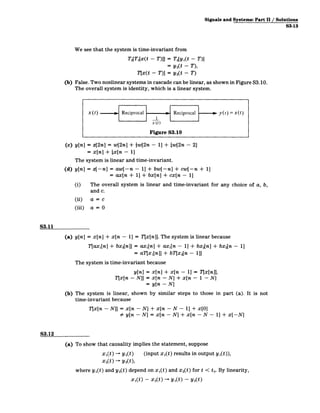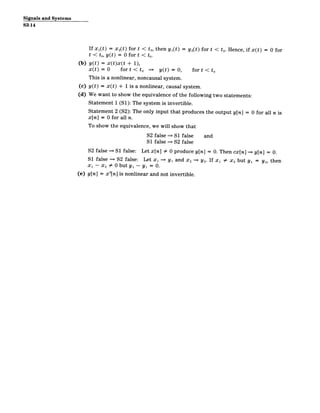Próximo SlideShare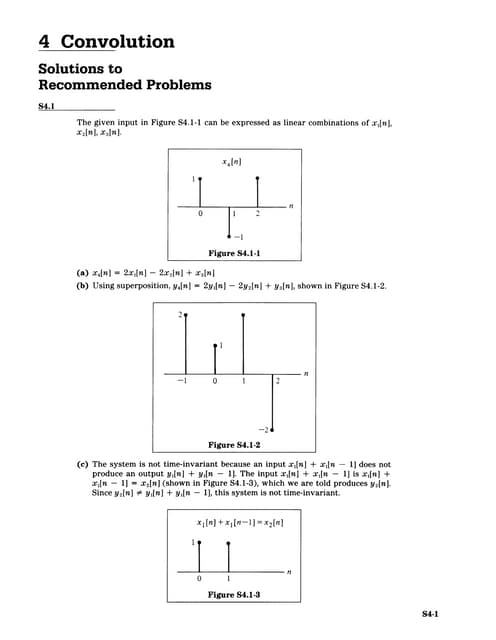convulution
Cargando en ... 3
1 de 15

### Signals and Systems part 2 solutions

1. 3 Signals and Systems: PartII Solutions to Recommended Problems S3.1 (a) x[n]= 8[n] + 8 [n - 3] n 0 1 2 3 Figure S3.1-1 (b) x[n] = u[n]-u[n-- 5] 1111 0041T -1 0 1 2 3 4 5 Figure S3.1-2 (c) x [n]=S[n] + -1 [n -- +(_1)2S[n] +(_1)3 5[n -3] 1 n -3 -2 -1 0 1 2 3 4 5 6 7 Figure S3.1-3 (d) x(t)= u(t +3) - u(t - 3) t -3 0 3 Figure S3.1-4 S3-1
2. Signals and Systems S3-2 (e) x(t) =6(t + 2) -2 0 Figure S3.1-5 t (f) S3.2 (1) (2) (3) (4) (5) (6) h d b e a, f None S3.3 (a) (b) x[n] = b[n - 1] - 26[n - 2] + 36[n - 3] s[n] = -u[n + 3] + 4u[n + 1] - 4u[n ­ - 26[n ­ 4] 2] + u[n - + b[n - 4] 5] S3.4 We are given Figure S3.4-1.
3. Signals and Systems: Part II / Solutions S3-3 x(- t) and x(1 - t) are as shown in Figures S3.4-2 and S3.4-3. x (-t) -12 Figure S3.4-2 x(1--t) x1-0 (a) -11 1 Figure S3.4-3 u(t + 1) - u(t - 2) is as shown in Figure S3.4-4. Hence, x(1 -1 - t)[u(t + 1) 0 1 2 Figure S3.4-4 - u(t - 2)]1looks as in Figure S3.4-5. t -1 5 6 1 Figure S3.4-5 t
4. Signals and Systems S3-4 (b) -u(2 - 3t) looks as in Figure S3.4-6. 2 3 t Hence, u(t + 1) Figure S3.4-6 - u(2 - 3t) is given as in Figure S3.4-7. 2 3 So x(1 ­ t)[u(t + Figure S3.4-7 1) - u(2 - 3t)] is given as in Figure S3.4-8. S3.5 (a) y[n] (b) y[n] (c) y[n] (d) y[n] = = = = x2[n] + x[n] - x2[n] + x[n] - H[x[n] ­ x[n - x2[n] + x 2 [n - G[x 2[n]] x2 [n] - X2 [n - x[n x[n 1]] 1] - 1 - 1] - 1] 2x[nJx[n ­ 1]
5. Signals and Systems: Part11/ Solutions S3-5 (e) y[n] = F[x[n] - x[n - 1]] = 2(x[n] - x[n - 1]) + (x[n - 1] - x[n - 2]) y[n] = 2x[n] - x[n - 1] - x[n - 2] (f) y[n] = G[2x[n] + x[n - 11] = 2x[n] + x[n - 11 - 2x[n - 1] - x[n - 21 = 2x[n] - x[n - 1] - x[n - 2] (a) and (b) are equivalent. (e) and (f) are equivalent. S3.6 Memoryless: (a) y(t) = (2 + sin t)x(t) is memoryless because y(t) depends only on x(t) and not on prior values of x(t). (d) y[n] = Ek=. x[n] is not memoryless because y[n] does depend on values of x[-] before the time instant n. (f) y[n] = max{x[n], x[n - 1], ... , x[-oo]} is clearly not memoryless. Linear: (a) y(t) = (2 + sin t)x(t) = T[x(t), T[ax1(t) + bx 2(t)] = (2 + sin t)[axi(t) + bx 2(tt) = a(2 + sin t)x 1(t) + b(2 + sin t)x 2(t) = aT[x1(t)] + bT[x 2(t)] Therefore, y(t) = (2 + sin t)x(t) is linear. (b) y(t) = x(2t) = T[x(t)], T[ax1(t) + bx 2(t)] = ax1(2t) + bx 2(t) = aT[x1 (t)) + bT[x 2(t)] Therefore, y(t) = x(2t) is linear. (c) y[n] = ( x[k] = T[x[n]], k=­ T[ax1 [n] + bx2[n]] = a T x1[k] + b L x 2[k] k= -x k=-w = aT[x1[n]] + bT[x2[n]] Therefore, yin] = E=_ x[k] is linear. (d) y[n] = > x[k] is linear (see part c). k= -o dxt d T[ax1(t) + bx 2(t)] = -[ax 1 (t)+±bx 2(t)] = a dx dt 1(t) + b dx dt 2(t) = aT[x1(t)] + bT[x2(t)] Therefore, y(t) = dx(t)/dt is linear. (f) y[n] = max{x[n], . . . , x[-oo]} = T1x[n]], T[ax1[n] + bx2[n]] = max{ax1[n] + bx 2[n], . . . , ax1[-oo] + bx2[ - o]} # a max{x[n], . . . , x1[-oo]} + b max{x 2[n], . . . , X2[-00]) Therefore, y[n] = max{x[n], .. . , x[-oo]} is not linear.
6. Signals and Systems S3-6 Time-invariant: (a) y(t) = (2 + sin t)x(t) = T[x(t)], T[x(t - T0 )] = (2 + sin t)x(t - T0 ) 9 y(t - T0) = (2 + sin (t - T0))x(t - T0) Therefore, y(t) = (2 + sin t)x(t) is not time-invariant. (b) y(t) = x(2t) = T[x(t)], T[x(t - T0 )] = x(2t - 2T0) # x(2t - TO) = y(t - T0 ) Therefore, y(t) = x(2t) is not time-invariant. (c) y[n] = ( x[kJ = T[x[n]], T[x[n - NO]J = ( x[k - NO] = y[n - N 0 ] Therefore, y[n] = Ek'= _.x[k] is time-invariant. (d) y[n] = E x[k] = T[x[n]], k=­ n n-NO T[x[n - NO]] = E x[k - N] = x[l] = y[n - N 0 ] k=- =-w0 Therefore, y[n] = E" _.x[k] is time-invariant. =dx(t) (e) y(t) dt T[x(t)], d T[x(t - To)] = x(t - To) = y(t - To) dt Therefore, y(t) = dx(t)/dt is time-invariant. Causal: (b) y(t) = x(2t), y(1) = x(2) The value of y(-) at time = 1 depends on x(-) at a future time = 2. Therefore, y(t) = x(2t) is not causal. (d) y[n] = ( x[k] k=­ Yes, y[n] = E .x[k] is causal because the value of y[-] at any instant n depends only on the previous (past) values of x[-]. Invertible: (b) y(t) = x(2t) is invertible; x(t) = y(t/2). (c) y[n] = E _.x[k] is not invertible. Summation is not generally an invertible operation. (e) y(t) = dx(t)/dt is invertible to within a constant. Stable: (a) If Ix(t) I < M, Iy(t) I < (2 + sin t)M.Therefore, y(t) = (2 + sin t)x(t) is stable. (b) If |x(t)| < M, |x(2t)I < M and ly(t)| < M. Therefore, y(t) = x(2t) is stable. (d) If |x[k]| 5 M, ly[n]j 5 M - E_,, which is unbounded. Therefore, y[n] = E"Lx[k] is not stable.
7. Signals and Systems: Part II / Solutions S3-7 S3.7 (a) Since H is an integrator, H-1 must be a differentiator. H~': y(t) dx(t) = d dt G: y(t) = x(2t) G 1: y(t) = x(t/2) (b) Solutions to Optional Problems S3.8 (a) x 2(t) = xi(t) - xi(t - 2) 2(t) = 1(t) y)(t - 2) Figure S3.8-1
8. Signals and Systems S3-8 (b) xA(t) = Xi( t) + xI(t + 1) y3(t)=y (t)+y 1 (t + 1) (c) x(t) = u(t - 1) -1 - u(t - 2) 10 1 2 Figure S3.8-2 y(t)=e-(t-1)u -1)+u(-t)+ u(t - 2) -u(1 - t) t (d) y[n] = 3y 1[n] ­ p 2y2[n] + 2 p14 Figure S3.8-3 2y3[n] 3 3 p n -3 -2 -1 0 1 2 3 -4 Figure S3.8-4
9. Signals and Systems: Part11/ Solutions S3-9 (e) y2[n] = y,[n] + yi[n - 11 y2 [n] 2 __ 0 1 2 3 4 5 Figure S3.8-5 Y3[n] = y 1 [n + 1] -1 0 2 3 4 Figure S3.8-6 (f) From linearity, y1(t) = 1r + 6 cos(2t) - 47 cos(5t) + '/e cos(6t), 1 + 0t 4" x 2(t) = 1 + t2 = (-t 2 )". n=O So y 2(t) = 1 - cos(2t) + cos(4t) - cos(6t) + cos(8t). S3.9 (a) (i) The system is linear because Tlaxi(t) + bx 2(t)] = 3 [ax1(t) + bx 2(t)](t - nT) n=­ = a T3 x1 (t)b(t - nT) + b ( xst - nT) = aT[xi(t)] + bT[x 2(t)] (ii) The system is not time-invariant. For example, let xi(t) = sin(22rt/T). The corresponding output yi(t) = 0. Now let us shift the input xi(t) by r/2 to get +r = cos (2) x 2 (t) = sin ( Now the output +00 Y2(0 >7 b(t - nT) =Ay, + 2= 0 n = -oo
10. Signals and Systems S3-10 (b) y(t) = E x(t)b(t - nT) = E cos(2,rt)b(t - nT) cos(27rt) 0< t Figure S3.9-1 T = 1 0 I ~11 tt 2 Figure S3.9-2
11. Signals and Systems: Part II / Solutions S3-11 y (t) T= T~__ t t -1 Figure S3.9-5 y(t) (c) y(t) = et cos(27rt)b(t Figure S3.9-6 - nT) etcos(2nrt) 22 Figure S3.9-7 et t T = I y (t) T=1 tt 1 Figure S3.9-8 3 2
12. Signals and Systems S3-12 y (t) T= Ie3 2 2 -e1/2 I I 2 2- I 3/2 t Figure S3.9-9 T= 4t y (t) YWe3 F r2 -el2 2 -e 3 12 Figure S3.9-10 T= Y3 12 1 y~t) 1 2 2 Figure S3.9-12 S3.10 (a) True. To see that the system is linear, write y2 (t) = T2 [T1 [x(t)]] T[x(t)], T1[ax1(t) + bx 2(t)] = aT1 [x1(t)] + bT[x2(t)] T2[T1[ax1(t) + bx 2 (t)]] = T2[aT1[x1(t)] + bT1[x 2(t)]I = aT2[T1[x1(t)]] + bT2[(T[x2t)]] = aT[x1(t)] + bT[x 2(t)J
13. Signals and Systems: Part 11/ Solutions S3-13 We see that the system is time-invariant from T2[T1 [x(t - T)]] = T2[y1(t - T)l = y 2(t -T), Tx(t - T)] = y 2(t - T) (b) False. Two nonlinear systems in cascade can be linear, as shown in Figure S3.10. The overall system is identity, which is a linear system. x(t) i Reciprocal - 1 x(t) Reciprocal 0 y(t)=x(t) Figure S3.10 (c) y[n] = z[2n] = w[2n] + {w[2n - 1] + {w[2n - 21 = x[n] + {x[n - 11 The system is linear and time-invariant. (d) y[n] = z[-nl = aw[-n ­ 11 + bw[-n] + cw[-n + 1] = ax[n + 11 + bx[nl + cx[n - 1] (i) The overall system is linear and time-invariant for any choice of a, b, and c. (ii) a= c (iii) a= 0 S3.11 (a) y[n] = x[n] + x[n - 11 = T[x[n]]. The system is linear because T[ax1[n] + bx2[n]| = ax1[n] + ax1[n - 1] + bx 2[n] + bx2[n - 1] = aT[x1 [n]] + bT[x 2[n - 1]] The system is time-invariant because y[n] = x[n] + x[n - 1] = Tjx[n]], T[x[n - N]] = x[n - N] + x[n ­ 1 - N] = y[n - N] (b) The system is linear, shown by similar steps to those in part (a). It is not time-invariant because T[x[n ­ N]] = x[n - N] # y[n - N] + x[n - N - 1] + x[O] = x[n ­ N] + x[n - N - 1] + x[-NJ S3.12 (a) To show that causality implies the statement, suppose x1(t) - yl(t) (input x1(t) results in output y1(t)), x 2(t) - y2), where y1(t) and y2(t) depend on x1(t) and x 2(t) for t < to. By linearity, xI(t) - x 2(t) -+ y 1(t) - y 2(t)
14. Signals and Systems S3-14 If Xi(t) = x 2(t) for t < to, then y1(t) = y2 (t) for t < to. Hence, if x(t) = 0 for t < to, y(t) = 0 for t < to. (b) y(t) = x(t)x(t + 1), x(t) = 0 for t < to =* y(t) = 0, for t < to This is a nonlinear, noncausal system. (c) y(t) = x(t) + 1 is a nonlinear, causal system. (d) We want to show the equivalence of the following two statements: Statement 1 (S1): The system is invertible. Statement 2 (S2): The only input that produces the output y[n] = 0 for all n is x[n] = 0 for all n. To show the equivalence, we will show that S2 false S1 false and S1 false S2 false S2 false == S1 false: Let x[n] # 0 produce y[n] = 0. Then cx[n] == y[n] = 0. S1 false S2 false: Let xi => yi and x 2 =* Y2. If x 1 # X2 but y1 = Y2, then X1 - X2 0 0 but yi - yi = 0. (e) y[n] = x 2 [n] is nonlinear and not invertible.
15. MIT OpenCourseWare http://ocw.mit.edu Resource: Signals and Systems Professor Alan V. Oppenheim The following may not correspond to a particular course on MIT OpenCourseWare, but has been provided by the author as an individual learning resource. For information about citing these materials or our Terms of Use, visit: http://ocw.mit.edu/terms.